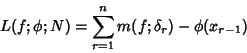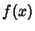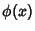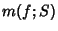## Darboux Integral

A variant of the Riemann Integral defined when the Upper and Lower Integrals, taken as limits of the Lower Sumand Upper Sumare equal. Here,is a Real Function,is a monotonic increasing function with respect to which the sum is taken,denotes the lower bound ofover the interval, anddenotes the upper bound.

See also Lower Integral, Lower Sum, Riemann Integral, Upper Integral, Upper Sum

References

Kestelman, H. Modern Theories of Integration, 2nd rev. ed. New York: Dover, p. 250, 1960.